Next: Discussion Up: Multiple subtraction Previous: Comparing the two methods

## Field data results

For this dataset, the choice of parameters such as filter sizes, patch sizes, number of iterations and Lagrange multipliers (e.g.,in equation ()) was determined by trial and error on small portions of the data for both methods. Experience shows that the Lagrange multipliers are the only parameters to be changed from one dataset to another.

Prestack results are presented in Figures,, andwhere panel (a) displays the input data, (b) the multiple model, (c) the estimated primaries with adaptive subtraction, and (d) the estimated primaries with the pattern-based approach. Figuredisplays a constant offset section at 500 m offset. The arrows in 1 and 2 point to locations where the multiple model is not accurate. At these locations, the pattern-based approach attenuates the multiples better than adaptive subtraction. Figuredisplays multiple removal results for one shot gather (X=664 km). Arrow 1 shows an area where the multiples are poorly predicted and poorly attenuated by both methods. At this location, the pattern-based techniques attenuates more multiples, however. Arrow 2 points to a diffracted event that is present in the multiple model but slightly shifted in time and offset. The adaptive subtraction is unable to remove this event while the pattern-based technique attenuates it. Arrow 3 shows another location where the multiples are better attenuated with the pattern-based approach. Note that some multiples, especially diffracted multiples, are still present in the estimated primaries because these events are totally absent in the model. Finally, Figuredisplays a shot gather where the multiple attenuation worked well for both techniques. This gather (X=693 km) comes from an area where the acquisition geometry and the geology are the most appropriate for the 2-D prediction, which explains why both techniques work very well. Arrow 1 points to one event that is still better preserved with the pattern-based method.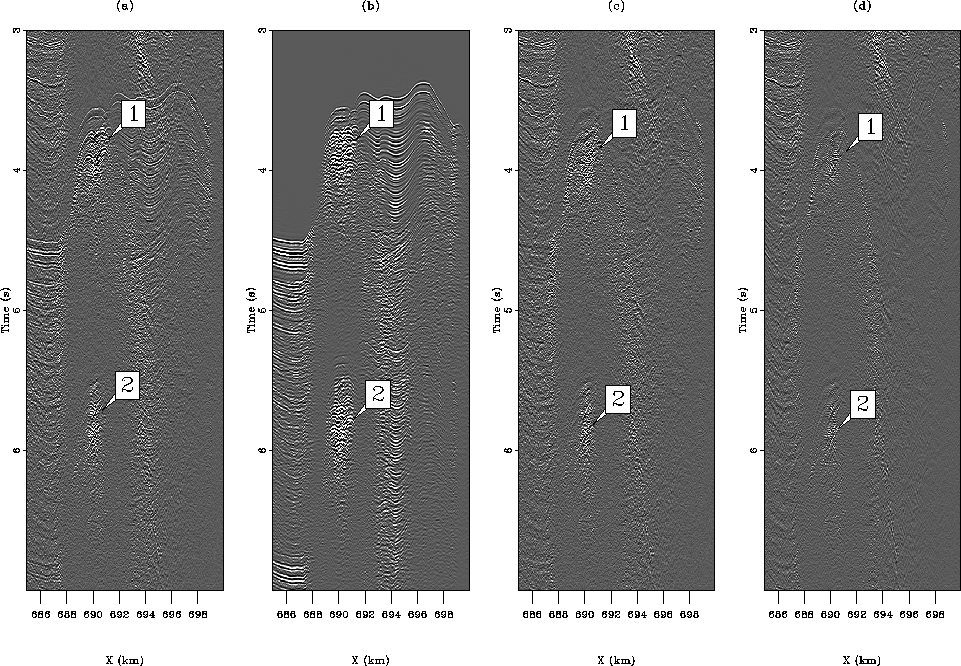window1-signal
Figure 12
A constant offset section at 500 m offset for (a) the input data, (b) the multiple model, (c) the estimated primaries with adaptive subtraction, and (d) the estimated primaries with the pattern-based approach. The horizontal axis represents the midpoint position. 1 and 2 points to area where the multiples are not accurately predicted and where the pattern-based approach performs better than adaptive subtraction.window3-signal
Figure 13
A shot gather (X=664 km) for (a) the input data, (b) the multiple model, (c) the estimated primaries with adaptive subtraction, and (d) the estimated primaries with the pattern-based approach. 1 shows multiples that are not properly predicted and not completely removed. 2 shows an event that is predicted at the wrong location but still attenuated in (d). 3 shows an area where the pattern-based approach attenuates more multiples than adaptive subtraction.window4-signal
Figure 14
A shot gather (X=693 km) for (a) the input data, (b) the multiple model, (c) the estimated primaries with adaptive subtraction, and (d) the estimated primaries with the pattern-based approach. 1 points to a primary that is better preserved in (d).Now, the data and the estimated primaries for both subtraction techniques are migrated with a split-step double square-root (DSR) migration algorithm (see Chapter). Figuresandshow a comparison of the migrated data (shown in (a)), the estimated primaries (shown in (b)), the estimated multiples (shown in (c)), and the multiple model from SRMP (shown in (d)) for the adaptive and pattern-based subtraction techniques, respectively. These results are close-ups of a sedimentary basin. The pattern-based approach (Figureb) yields cleaner multiple-free panels. In addition, the multiples inside the circle are better attenuated with the pattern-based approach than with the adaptive-subtraction technique (Figureb). One reason for these differences comes from the modeling inaccuracies of 2-D SRMP, as exemplified in Figuresc andc. There, it appears that modeled multiples do not match the true multiples in the migrated data exactly (e.g., Figurea). The pattern-based approach is able to cope better with the differences between true and modeled multiples.

Now, Figuresanddisplay multiple attenuation results in the presence of a salt body. Again, the base of salt reflection is cleaner and more continuous with the pattern-based approach (Figureb) than with adaptive subtraction (Figureb). Figuresd andd show the migrated multiple model. The model exhibits important kinematic differences with the true multiples. For instance, the water-bottom multiple at 4.2 km is slightly shifted horizontally in the model. The pattern-based approach is able to remove this multiple albeit modeling errors.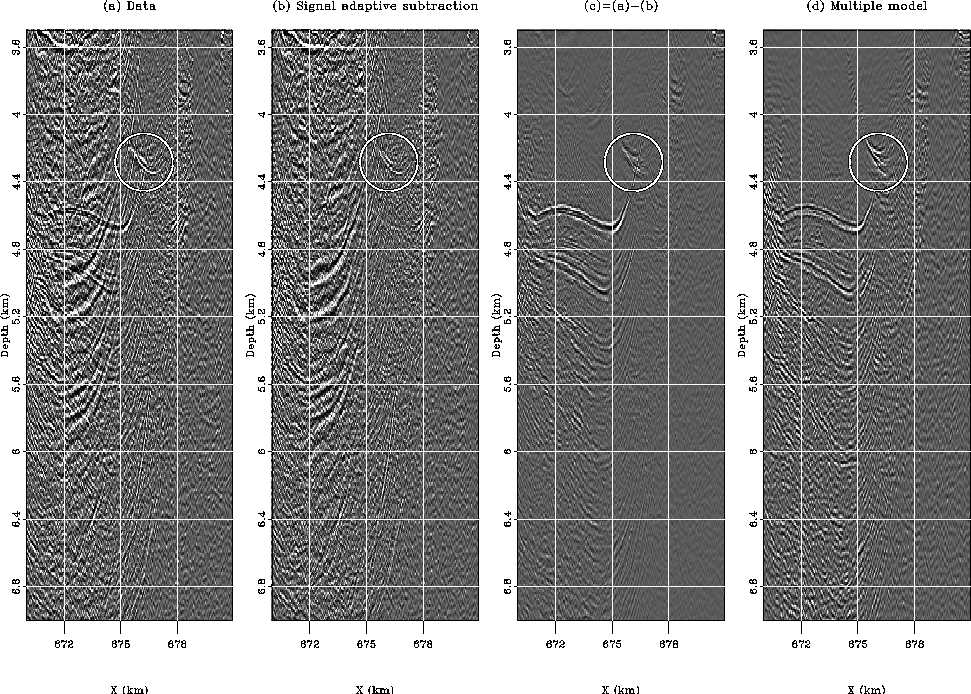Figure 15
Migrated images for (a) the data, (b) the estimated primaries with adaptive subtraction, (c) the difference between (a) and (b), i.e., the estimated multiples, and (d) the multiple model with SRMP. Multiples in the circle are not well attenuated.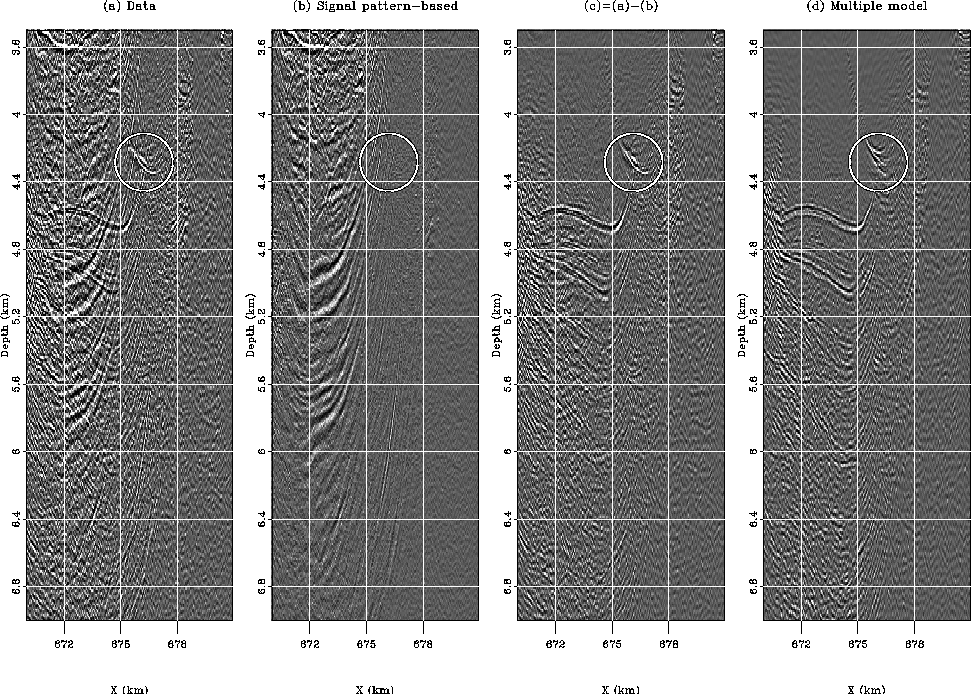mig_pattern
Figure 16
Migrated images for (a) the data, (b) the estimated primaries with the pattern-based approach, (c) the difference between (a) and (b), i.e., the estimated multiples, and (d) the multiple model with SRMP. Multiples in the circle are better attenuated in (b) than in Figureb. In addition, the primaries look more continuous everywhere.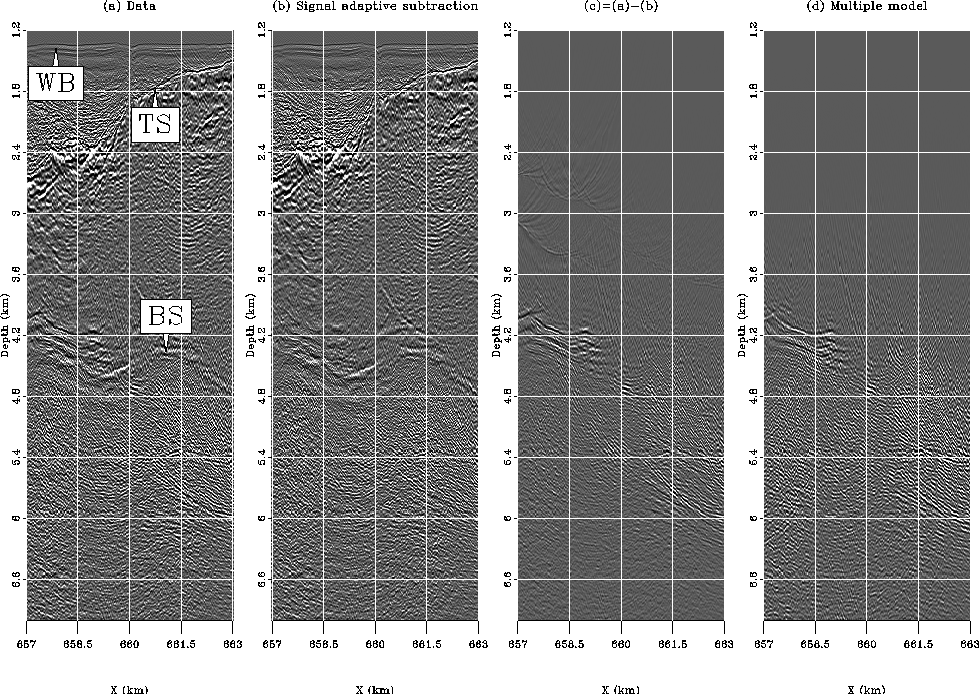Figure 17
Migrated images for (a) the data, (b) the estimated primaries with adaptive subtraction, (c) the difference between (a) and (b), i.e., the estimated multiples, and (d) the multiple model with SRMP. The base of salt is indicated by an arrow in (a). Some subsalt multiples are still present. Arrow WB shows the water bottom, TS the top of salt, and BS the base of salt.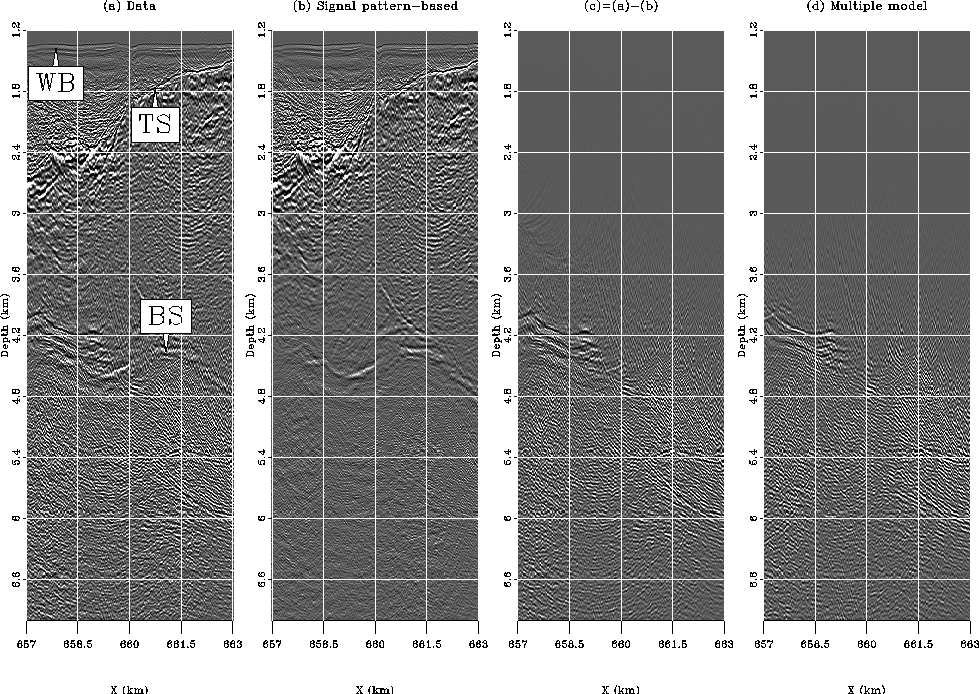mig_pattern1
Figure 18
Migrated images for (a) the data, (b) the estimated primaries with the pattern-based approach, (c) the difference between (a) and (b), i.e., the estimated multiples, and (d) the multiple model with SRMP. The base of salt is better preserved than in Figureb and the subsalt multiples are well attenuated. Arrow WB shows the water bottom, TS the top of salt, and BS the base of salt.Finally, angle-domain common image gathers (ADCIG) are created with the method of Sava and Fomel (2003) after migration Stolt and Weglein (1985); Weglein and Stolt (1999). Figuresa,b, andc show three ADCIG for the data, the estimated primaries with adaptive subtraction, and the estimated primaries with the pattern-based approach, respectively. This ADCIG is located within the salt boundaries. The primary at 4.6 s. is the base of salt reflection. Events with large curvatures in Figureb are multiples. Again, the pattern-based approach produces a better panel. The method of Sava and Guitton (2005) could be used to remove the remaining multiples with a Radon-based technique. As a final example, Figureshow a comparison of ADCIGs outside the salt body, within the sedimentary basin of Figuresand. The primaries are more continuous and better preserved with the pattern-based approach (Figureb) than with adaptive subtraction (Figurec).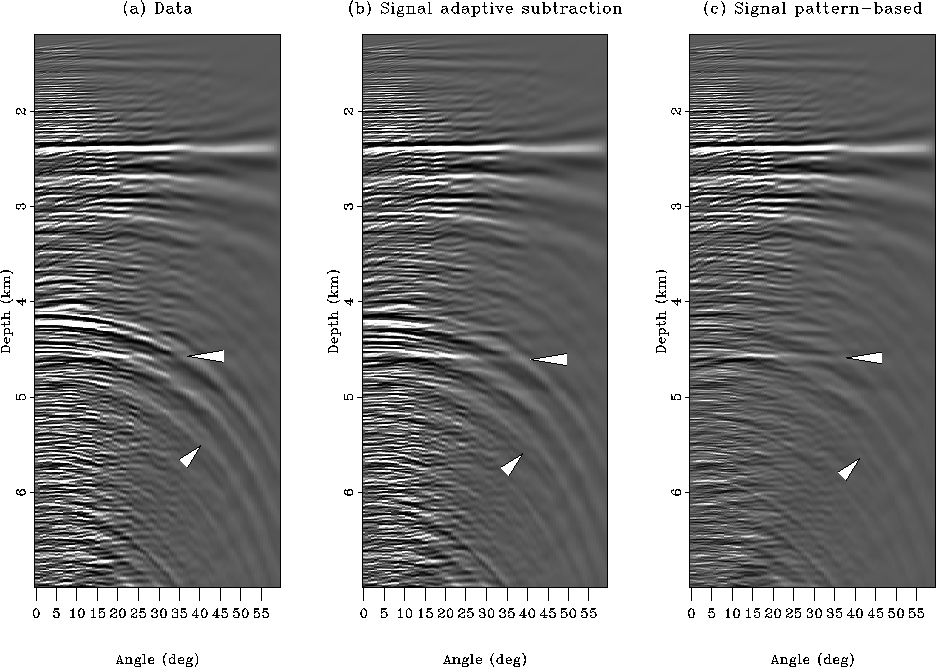ang1
Figure 19
ADCIG at X=658.975 for (a) the data, (b) the estimated primaries with adaptive subtraction, and (c) the estimated primaries with the pattern-based approach. The arrow at 4.6 s. shows the base of salt reflection. The second arrow shows multiples at high angles with strong curvatures. The pattern-based approach yields a cleaner gather.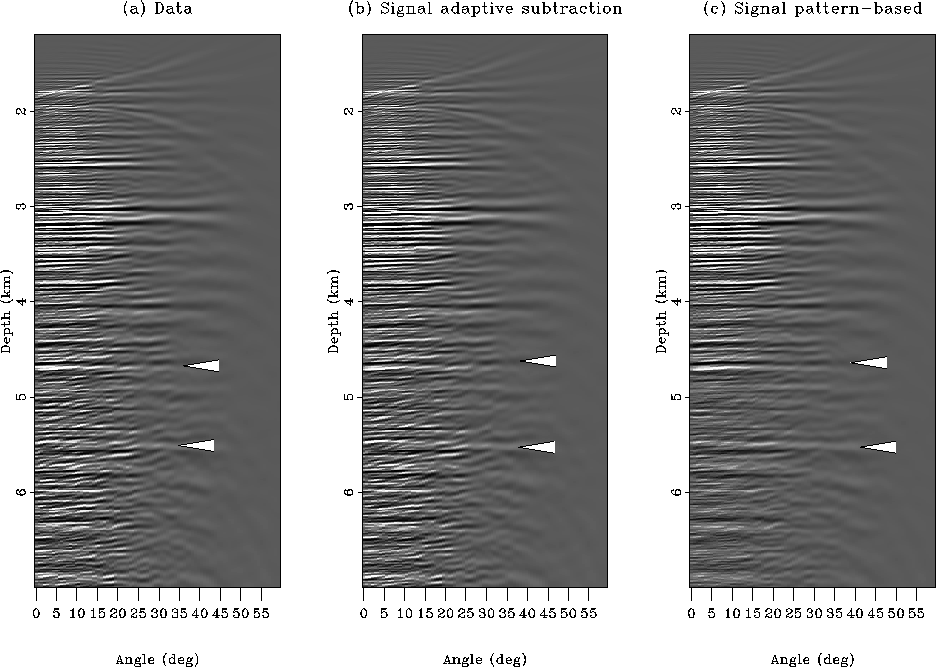ang2
Figure 20
ADCIG at X=674.325 for (a) the data, (b) the estimated primaries with adaptive subtraction, and (c) the estimated primaries with the pattern-based approach. The two arrows show primaries that are more continuous after pattern-based subtraction of the multiples.Next: Discussion Up: Multiple subtraction Previous: Comparing the two methods
Stanford Exploration Project
5/5/2005